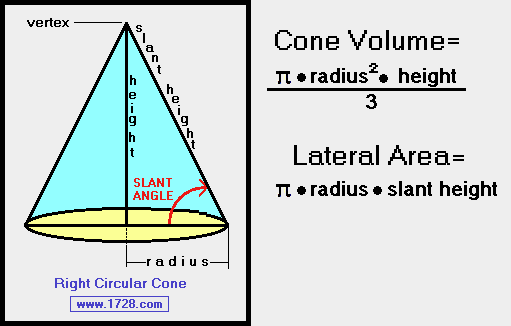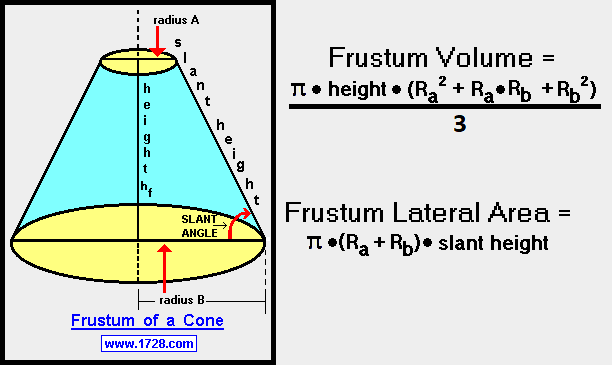Right Circular Cone Calculator & Cone Frustum Calculator Scroll down for instructions and definitions This calculator was extensively rewritten on November, 2017. It can now solve for cones if you know 2 out of these 4 variables: RADIUS     HEIGHT     SLANT HEIGHT     SLANT ANGLE First choose cone or frustum: cone frustum Then, if you chose cone, select the 2 data items you do know. (These buttons work ONLY with cones and NOT frustums.) Radius & Height Height & Slant Height Height & Slant Angle Slant Height & Radius Slant Height & Slant Angle Radius & Slant Angle Right Circular ConesA right circular cone is a geometric solid formed by all lines drawn from a circular base and terminating at a common point called the vertex, which is located above the center of the circular base. A perpendicular line drawn from the vertex to the center of the base is called the height of the cone. The formula for finding circular area has already been discussed on this site as well as the Pythagorean Theorem which can be used to calculate slant height. Cone Volume = (π  r²  h) ÷ 3 Lateral Area = (π  r  slant height) Frustum of a ConeA frustum (sometimes incorrectly spelled frustrum) of a right circular cone is that part of a right circular cone between the base of the cone and a plane which intersects the cone parallel to the base. There are formulas for frustums, but if you think about it, the calculations can be done by extending the frustum height to a vertex and then subtracting the "upper" cone's values from the complete (or extended) cone yielding the values for the frustum. If we call 'Radius A' Ra 'Radius B' Rband the frustum height hf then the "upper cone height" hu is found by: hu = (hf  Ra) ÷ (Rb - Ra) and the height of the extended cone is (hu + hf). From there you can calculate the volumes and areas of each cone and subtract the upper cone values from the extended cone to find the frustum values. For those who'd still like to see the frustum formulas, here they are: Frustum Volume = (π  height  (Ra2 + Ra  Rb + Rb2) ÷ 3) Frustum Lateral Area = (π  (Ra + Rb)  slant height) Yes you could use these formulas for cone calculations. However, as you know, here at "1728", there's always a calculator to do the work for you. Significant Figures >>> The default setting is for 5 significant figures but you can change that by inputting another number in the box above. Answers are displayed in scientific notation and for easier readability, numbers between .001 and 1,000 will be displayed in standard format (with the same number of significant figures.) The answers should display properly but there are a few browsers that will show no output whatsoever. If so, enter a zero in the box above. This eliminates all formatting but it is better than seeing no output at all. Return To Geometry Index Return To Home Page Copyright © 1999 - 1728 Software Systems